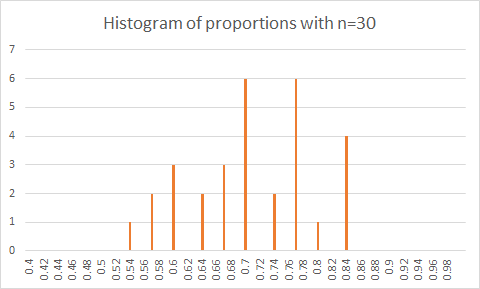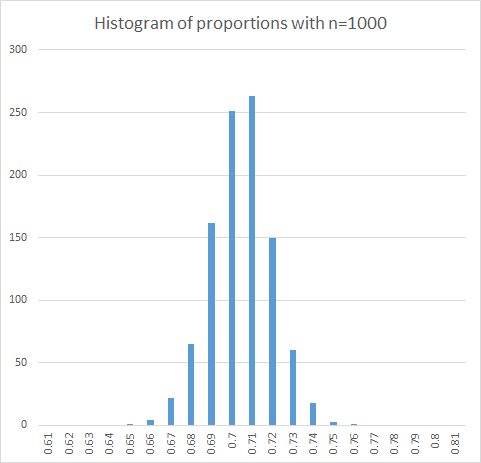28
Aug 16

## The pearls of AP Statistics 23

The law of large numbers: the mega delusion of AP Statistics

### The gist

They say: Figure 7.8 displays sampling distributions of the sample mean x for four different shapes for the population distribution from which samples are taken. The population shapes are shown at the top of the figure; below them are portrayed the sampling distributions of x for random sampling of sizes n = 2, 5, and 30. Even if the population distribution itself is uniform (column 1 of the figure) or U-shaped (column 2) or skewed (column 3), the sampling distribution of the sample mean has approximately a bell shape when n is at least 30 and sometimes for n as small as 5. In practice, the sampling distribution is usually close to bell shape when the sample size n is at least 30 (Agresti and Franklin, p.321)

I say: The original distributions in Figure 7.8 are all continuous. If they are discrete (below I consider the coin as an example), the picture is much worse. Besides, Figure 7.8 illustrates theoretical sampling distributions. However, usually a sample from a nice distribution behaves much worse than the shape of the distribution predicts. Based on the last line of Figure 7.8, AP Statistics texts in unison recommend using sample sizes 30 or larger.

The standard requirement in professional statistical journals is that authors have to confirm their theoretical statements with Monte Carlo (computer) simulations. The commonly used sample sizes are from several hundred to 10,000. This shows how slow the rate of convergence in the laws of large numbers and central limit theorems is. Even Agresti and Franklin in some of their exercises suggest simulating 10,000 times. The Merriam-Webster definition of delusion is "something that is falsely or delusively believed or propagated". Yes, the rule of thumb of 30 is delusively believed AND falsely propagated.

### Coin simulationsFigure 1. Histogram of proportions with n=30Figure 2. Histogram of proportions with n=100Figure 3. Histogram of proportions with n=1000

Figure 1 shows the histogram of proportions of 1 with n=30, Figure 2 shows the same with a larger sample size n=100 and in Figure 3 the sample size is increased to n=1000. Visual impressions are subjective. To me, the histogram for n=30 does not look like coming from a normal distribution. The one for n=1000 certainly does.

### Illustrations in Excel

In your class you can use my Excel file with simulations. The file contains macros. In Options/Trust Center/Trust Center Settings/Message Bar click "Show the message bar". When you open the file and see the security warning about active content, click Enable content. On worksheets named "n=30", "n=100", and "n=1000" there are command buttons named "Resample". Press the button and you will see the histogram renewed after each resampling. On the first two worksheets for n=30 and n=100 this is done very quickly. On the page "n=1000" resampling on my computer takes 2-3 seconds. The worksheet "LLN" contains graphical illustrations of the law of large numbers for sample sizes up to 100 and 1000, respectively.

If you want to see my macros, in Options/Customize Ribbon/Main Tabs enable the Developer tab (which by default is hidden) and click OK. When you are on a page with Resample button, click View Code on the Developer tab. This will take you to the Visual Basic Editor.